Test: Theory of Random Variable & Noise

# Test: Theory of Random Variable & Noise - Electronics and Communication Engineering (ECE)

Test Description

## 10 Questions MCQ Test GATE ECE (Electronics) 2023 Mock Test Series - Test: Theory of Random Variable & Noise

Test: Theory of Random Variable & Noise for Electronics and Communication Engineering (ECE) 2023 is part of GATE ECE (Electronics) 2023 Mock Test Series preparation. The Test: Theory of Random Variable & Noise questions and answers have been prepared according to the Electronics and Communication Engineering (ECE) exam syllabus.The Test: Theory of Random Variable & Noise MCQs are made for Electronics and Communication Engineering (ECE) 2023 Exam. Find important definitions, questions, notes, meanings, examples, exercises, MCQs and online tests for Test: Theory of Random Variable & Noise below.
Solutions of Test: Theory of Random Variable & Noise questions in English are available as part of our GATE ECE (Electronics) 2023 Mock Test Series for Electronics and Communication Engineering (ECE) & Test: Theory of Random Variable & Noise solutions in Hindi for GATE ECE (Electronics) 2023 Mock Test Series course. Download more important topics, notes, lectures and mock test series for Electronics and Communication Engineering (ECE) Exam by signing up for free. Attempt Test: Theory of Random Variable & Noise | 10 questions in 30 minutes | Mock test for Electronics and Communication Engineering (ECE) preparation | Free important questions MCQ to study GATE ECE (Electronics) 2023 Mock Test Series for Electronics and Communication Engineering (ECE) Exam | Download free PDF with solutions
 1 Crore+ students have signed up on EduRev. Have you?
Test: Theory of Random Variable & Noise - Question 1

### A certain random variable has the CDF (cumulative distribution function) given by: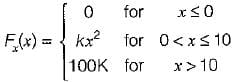Which of the following options is/are not correct?

Detailed Solution for Test: Theory of Random Variable & Noise - Question 1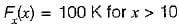Since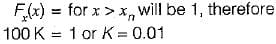Now,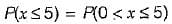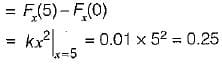Also,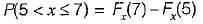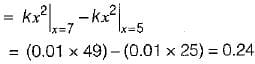Hence, option (c) is not correct.

Test: Theory of Random Variable & Noise - Question 2

### A radio receiver with 10 kHz bandwidth has a noise figure of 30 dB. The noise power at input is (take K = 1.38 x 10-23 J/Kelvin and assume room temperature of 300 K)

Detailed Solution for Test: Theory of Random Variable & Noise - Question 2

Noise figure,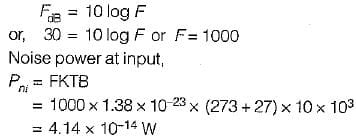Test: Theory of Random Variable & Noise - Question 3

### The probability density function of a random variable is given by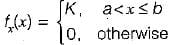The value of constant K is

Detailed Solution for Test: Theory of Random Variable & Noise - Question 3

Since the area under the probability density function is equal to 1, therefore.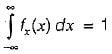or,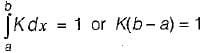or,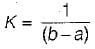Test: Theory of Random Variable & Noise - Question 4

Consider the following statements associated with different types of noise occurring in nature:
1. The flicker noise occurs at high operating frequencies (few MHz).
2. Shot noise is produced due to random variations in the arrival of electrons at the output electrode of an amplifying device.
3. The thermal noise is proportional to temperature and the value of the resistance and is independent of bandwidth.
4. Noise can also be represented by fictitious temperature called noise temperature.
Which of the statements given above is/are not correct?

Detailed Solution for Test: Theory of Random Variable & Noise - Question 4
• The flicker noise occurs at low operating frequencies (few KHz). Hence, statement-1 is not correct.
• The thermal noise voltage is given by,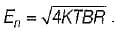Hence, it is proportional to temperature, bandwidth and the value of resistance. Thus, statement-3 is not correct.
• Statements 1 and 4 are correct.
Test: Theory of Random Variable & Noise - Question 5

Over a certain binary communication channel (BCC), symbol ‘O' is transmitted with a probability of 0.4 and symbol ‘1’ is transmitted with a probability of 0.6. The probability of error when '1' is transmitted is 10-4 and that when a ‘0' is transmitted is 10-6.
What is the probability of error in the channel?

Detailed Solution for Test: Theory of Random Variable & Noise - Question 5

BCC with different probabilities: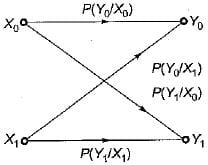According to question,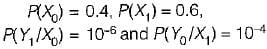Here,  P(Y0/X0) =  Probability of error when ‘1 ’ is transmitted
P(Y1/X0) = Probability of error when ‘0 ’ is transmitted
∴ Probability of error in channel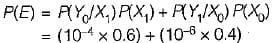or, P(E) = 0.604 x 10-4

Test: Theory of Random Variable & Noise - Question 6

Thermal noise is also known as

Detailed Solution for Test: Theory of Random Variable & Noise - Question 6

The electronic components generate thermal, shot noise. The thermal noise is also called Johnson noise.

Test: Theory of Random Variable & Noise - Question 7

The joint PDF of the.random variable x and y is given by,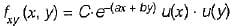where, a and b are constants. The value of C is

Detailed Solution for Test: Theory of Random Variable & Noise - Question 7

The joint CDF (cumulative distribution function ) is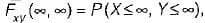which will be equal to 1 because the probability of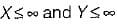covers all the possible values of random variables X and Y.
∴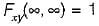According to the definition of CDF, we have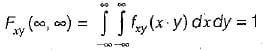Substituting the value of PDF, we have: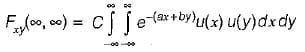or,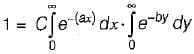or,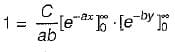or,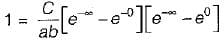or,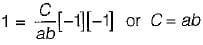Test: Theory of Random Variable & Noise - Question 8

Assertion (A): if the random process x(t) changes very rapidly with time, the autocorrelation function will decrease rapidly to zero.
Reason (R): The autocorrelation function Rx(τ) is a measure of interdependence of two random variables obtained by observing the random process x(t) at time τ seconds apart.

Detailed Solution for Test: Theory of Random Variable & Noise - Question 8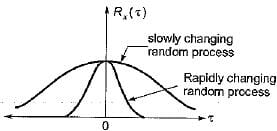From above figure we can conclude that both assertion and reason are true and reason is the correct explanation of assertion.

Test: Theory of Random Variable & Noise - Question 9

Consider the following statements associated with conditional probability density function (PDF):
1. The conditional PDF is basically a probability, density function (PDF).
2. The conditional PDF is a non-negative function.
3. The area under a conditional PDF is always equal to 1,
4. The conditional density functions reduce to the marginal density functions.
Which of the statements given above are correct?

Detailed Solution for Test: Theory of Random Variable & Noise - Question 9

• The conditional PDF is basically a PDF Since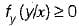i.e. the conditional PDF is a non ­negative function.
Hence, statements 1 and 2 are correct.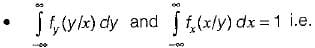th e area under a conditional PDF is always equal to 1. Hence, statement-3 is also correct.
• If the random variables x and y are statistically independent, then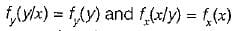This means that the conditional density functions reduce to the marginal density functions. Hence, statement-4 is also correct.

Test: Theory of Random Variable & Noise - Question 10

The distribution function of random variable is

Detailed Solution for Test: Theory of Random Variable & Noise - Question 10

The distribution function of a random variable is the probability that the value taken by the random variable is less than or equal to the real number x.

## GATE ECE (Electronics) 2023 Mock Test Series

21 docs|263 tests
Information about Test: Theory of Random Variable & Noise Page
In this test you can find the Exam questions for Test: Theory of Random Variable & Noise solved & explained in the simplest way possible. Besides giving Questions and answers for Test: Theory of Random Variable & Noise, EduRev gives you an ample number of Online tests for practice

## GATE ECE (Electronics) 2023 Mock Test Series

21 docs|263 tests(Scan QR code)